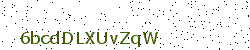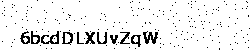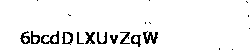# Captcha me if you can

Published on Sunday, 13 January 2013 in Python ; tagged with python, captcha, ocr, pytesser, tesseract ; text version

I've always wondering how can the bots on the internet crack captchas. How can a algorithm read an image.
Then I heard about OCRs (Optical Character Recognition) which are developped in order to do such tasks.

The captcha below will be our example:After creating a kind of database of the letters, the result was clearly bad. I mean I had 0 cracked captcha...
The main fact is that I assumed the text was horizontal but it's not always the case.
Moreover, the space between the letters is really thin sometimes.
So when the algorithm was trying to split the letters, some of them were gathered by 4.

# PyTesser, I choose you!

Then I looked for an OCR and I found Tesseract, an engine from Google. It is described as the best free OCR at this time.
I want quick results so I use PyTesser, a kind of Python binding of Tesseract.
Now it's time to try.

```import os
import sys
import re
from PIL import Image
from libtesserwrap import Tesserwrap

if __name__ == "__main__":
for arg in sys.argv[1:]:
if not os.path.isfile(arg):
print arg, 'cant be found.'
sys.exit(1)
else:
im = Image.open(arg)
im = im.convert("P")
ocr = Tesserwrap()
im.tostring(),
1,
im.size,
0,
0,
im.size,
im.size
)
# In our case, the captcha only contains 12 alphanum characters
else:
```

If we try it on our captcha, the result is:

```\$ python2.7 main.py captcha_test.gif
String found: 6bcdDLXUvZqW
```

Of course I choose a captcha where Tesseract finds the good result.
In order not to fool you, I runned the script on 1000 captchas and see how many of them it can read.
We have in our case an accuracy of 3% (30 over 1000), which is something at least...

# Improve the accurary

Well, 3% is something but it's not really accurate.
I want to know if I can improve the result by pre-processing the captcha.
I first converted the captcha into a black and white image and then I deleted the isolated pixels.

## Black and white

According to the article decoding captcha's, I check the colors of the captcha to get the most used.
Since the captcha is generated with several colors, it was easier to get the background one. In our case it's 225.

```def convert_black_and_white(im):
"""Convert the captcha in black and white."""

im2 = Image.new("P", im.size, 255)
for x in xrange(im.size):
for y in xrange(im.size):
pix = im.getpixel((y, x))
if pix == 225:
im2.putpixel((y, x), 255)
else:
im2.putpixel((y, x), 0)
return im2
```## Delete isolated pixels

The algorithm here is really simple. We check each white pixel and if its neighbours are black, we delete it.

```def delete_noise(im):
"""Delete isolated pixels on an image."""

for y in xrange(1, im.size - 1):
for x in xrange(1, im.size - 1):
pix = im.getpixel((y, x))
if pix != 255:
border = [
im.getpixel((y + 1, x - 1)),
im.getpixel((y + 1, x)),
im.getpixel((y + 1, x + 1)),
im.getpixel((y, x - 1)),
im.getpixel((y, x + 1)),
im.getpixel((y - 1, x - 1)),
im.getpixel((y - 1, x)),
im.getpixel((y - 1, x + 1))
]
if not 0 in border:
im.putpixel((y, x), 255)
return im
```